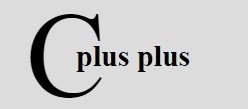# C++ Stack

A stack is a LIFO (Last In First Out) structure and physically it can be implemented as an array or as a linked list. A stack implemented as an array inherits all the properties of an array and if implemented as a linked list, then all the characteristics of a linked list are possessed by it. But whatever way a stack may be implemented, insertions and deletions occur at the top only. An insertion in a stack is called pushing and a deletion from a stack is called popping.

## Array Stack

As arrays are static data structures, i.e., space required for them must be predetermined i.e., how many total elements will be existing together at any point of time must be known beforehand. Therefore, creation of a stack as array involves determining the number of elements beforehand.

### Insertion in a Stack as an Array

Pushing an element in the stack may involve shifting of elements (depending upon how you implemented it) as the new element will be inserted at the top only. For instance, at any point of time we have stack (as an array) like Fig.1(a).

After pushing P, stack becomes as Fig.1(b). After pushing another element L, the stack becomes as Fig.1(c).

Fig.1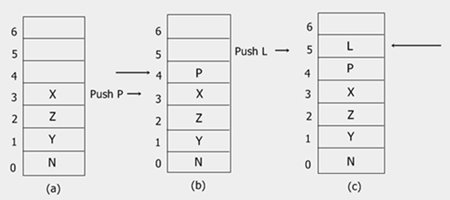In case the array is full and no new element can be accommodated, it is called STACK-FULL condition. This condition is also called an OVERFLOW.

### Deletion in a Stack as an Array

Popping i.e., deletion of an element from a stack removes the element at top most position. As you seen the earlier figure of pushing, for the popping the figure is same but it is in opposite manner i.e., after popping an element, it will removed.

A linked stack is a dynamic data structure where space requirements need not be predetermined. A stack implemented as a linked list also inherits all these properties. The creation of stack (as a linked list) is the same as the creation of a linked list i.e., after getting a node for the ITEM to be inserted, TOP (pointer pointing to the top) points to the newly inserted node.

### Insertion in a Linked Stack

As a push can only occur at the top, TOP gets modified every time.

### Deletion from a Linked Stack

Deletion i.e., popping also requires modification of TOP i.e., TOP is made to point to the next node in the sequence.

## C++ Stack Example

Here are some example program demonstrating the concept of stack in C++ practically.

## Pushing

As already told, insertion in a stack is called as pushing.

#### Pushing in Stack-Array

```/* C++ Stack - Example Program of C++ Stack
* This C++ program demonstrates the concept
* of Pushing in the stack-array in C++ */

#include<iostream.h>
#include<stdlib.h>
#include<conio.h>

int push(int [], int &, int);
void display(int [], int);
const int SIZE = 50;

void main()
{
clrscr();
int stack[SIZE], item, top=-1, res;
char ch='y';
while(ch=='y' || ch=='Y')
{
cout<<"Enter item for insertion: ";
cin>>item;
res = push(stack, top, item);
if(res == -1)
{
cout<<"Overflow..!!..Aborting..Press a key to exit..\n";
getch();
exit(1);
}
cout<<"Element inserted successfully..!!\n";
cout<<"\nThe Stack now is:\n";
display(stack, top);
cout<<"\nWant to enter more ? (y/n).. ";
cin>>ch;
}
getch();
}

int push(int stack[], int &top, int elem)
{
if(top == SIZE-1)
{
return -1;
}
else
{
top++;
stack[top] = elem;
}
return 0;
}
void display(int stack[], int top)
{
cout<<stack[top]<<" <-- "<<"\n";
for(int i=top-1; i>=0; i--)
{
cout<<stack[i]<<"\n";
}
}```

Here is the sample output of the above C++ program: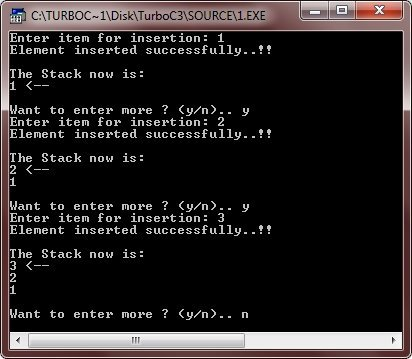```/* C++ Stack - Example Program of C++ Stack
* This C++ program demonstrates the concept
* of Pushing in the linked-stack in C++ */

#include<iostream.h>
#include<stdlib.h>
#include<conio.h>

struct node
{
int info;
node *next;
} *top, *newptr, *save, *ptr;

node *create_new_node(int);
void push(node *);
void display(node *);

void main()
{
clrscr();
int inf;
char ch='y';
top=NULL;
while(ch=='y' || ch=='Y')
{
cout<<"Enter information for the new node.. ";
cin>>inf;
newptr = create_new_node(inf);
if(newptr == NULL)
{
cout<<"\nSorry..!!..Cannot create new node..!!..Aborting..!!\n";
cout<<"Press any key to exit..\n";
getch();
exit(1);
}
push(newptr);
display(top);
cout<<"\nWant to enter more ? (y/n).. ";
cin>>ch;
}
getch();
}

node *create_new_node(int x)
{
ptr = new node;
ptr->info = x;
ptr->next = NULL;
return ptr;
}

void push(node *n)
{
if(top==NULL)
{
top=n;
}
else
{
save = top;
top = n;
n->next = save;
}
}

void display(node *n)
{
while(n != NULL)
{
cout<<n->info<<" -> ";
n = n->next;
}
cout<<"!!\n";
}```

Here is the sample output of this C++ program: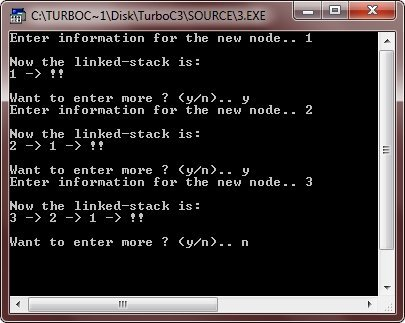## Popping

As already told, deletion from a stack is called as popping.

#### Popping from Array-Stack

```/* C++ Stack - Example Program of C++ Stack
* This C++ program demonstrates the concept
* of Popping from the stack-array in C++ */

#include<iostream.h>
#include<stdlib.h>
#include<conio.h>

int pop(int [], int &);
int push(int [], int &, int);
void display(int [], int);
const int SIZE = 50;

void main()
{
clrscr();
int stack[SIZE], item, top=-1, res;
char ch='y';
while(ch=='y' || ch=='Y')
{
cout<<"Enter item for insertion: ";
cin>>item;
res = push(stack, top, item);
if(res == -1)
{
cout<<"Overflow..!!..Aborting..Press a key to exit..\n";
getch();
exit(1);
}
cout<<"\nThe Stack now is:\n";
display(stack, top);
cout<<"\nWant to enter more ? (y/n).. ";
cin>>ch;
}
cout<<"Now the deletion of elements starts..\n";
ch='y';
while(ch=='y' || ch=='Y')
{
res = pop(stack, top);
if(res==-1)
{
cout<<"\nUnderflow..!!..Aborting..!!..Press a key to exit..\n";
getch();
exit(2);
}
else
{
cout<<"\nElement deleted is: "<<res<<endl;
cout<<"\nThe Stack now is:\n";
display(stack, top);
}
cout<<"Want to delete more ? (y/n).. ";
cin>>ch;
}
getch();
}

int push(int stack[], int &top, int elem)
{
if(top == SIZE-1)
{
return -1;
}
else
{
top++;
stack[top] = elem;
}
return 0;
}

int pop(int stack[], int &top)
{
int ret;
if(top==-1)
{
return -1;
}
else
{
ret=stack[top];
top--;
}
return ret;
}

void display(int stack[], int top)
{
if(top==-1)
{
return;
}
cout<<stack[top]<<" <-- "<<"\n";
for(int i=top-1; i>=0; i--)
{
cout<<stack[i]<<"\n";
}
}```

Here are some sample output of the above C++ program: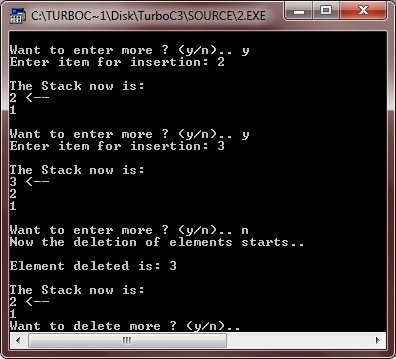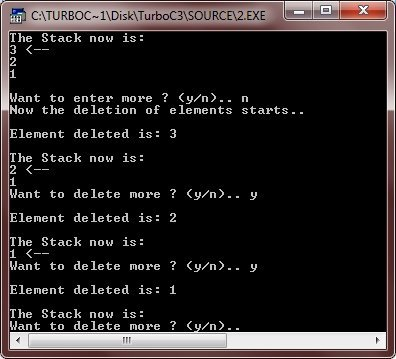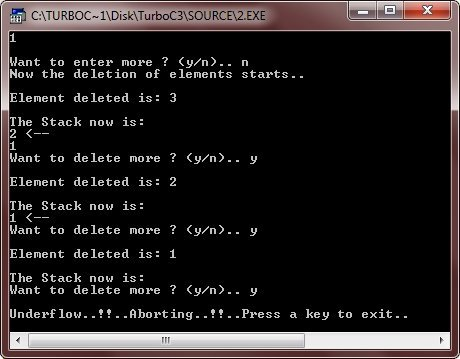```/* C++ Stack - Example Program of C++ Stack
* This C++ program demonstrates the concept
* of Popping from the linked-stack in C++ */

#include<iostream.h>
#include<stdlib.h>
#include<conio.h>

struct node
{
int info;
node *next;
} *top, *newptr, *save, *ptr;

node *create_new_node(int);
void push(node *);
void pop();
void display(node *);

void main()
{
clrscr();
int inf;
char ch='y';
top=NULL;
while(ch=='y' || ch=='Y')
{
cout<<"Enter information for the new node.. ";
cin>>inf;
newptr = create_new_node(inf);
if(newptr == NULL)
{
cout<<"\nSorry..!!..Cannot create new node..!!..Aborting..!!\n";
cout<<"Press any key to exit..\n";
getch();
exit(1);
}
push(newptr);
cout<<"\nWant to enter more ? (y/n).. ";
cin>>ch;
}
clrscr();
do
{
cout<<"The Stack now is: \n";
display(top);
cout<<"\nWant to pop an element ? (y/n).. ";
cin>>ch;
if(ch=='y' || ch=='Y')
{
pop();
}
cout<<"\n";
}while(ch=='y' || ch=='Y');

getch();
}

node *create_new_node(int x)
{
ptr = new node;
ptr->info = x;
ptr->next = NULL;
return ptr;
}

void push(node *n)
{
if(top==NULL)
{
top=n;
}
else
{
save = top;
top = n;
n->next = save;
}
}

void pop()
{
if(top==NULL)
{
cout<<"\nUnderflow..!!..Press any key to exit..\n";
getch();
exit(2);
}
else
{
ptr = top;
top = top->next;
delete ptr;
}
}

void display(node *n)
{
while(n != NULL)
{
cout<<n->info<<" -> ";
n = n->next;
}
cout<<"!!\n";
}```

Here are some sample runs of the above C++ program: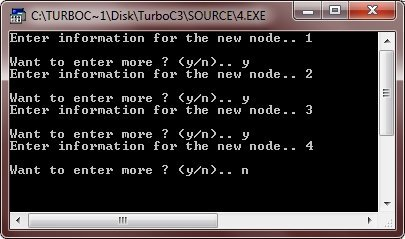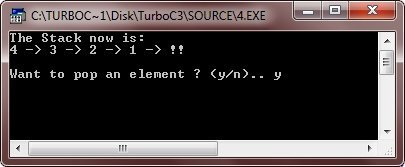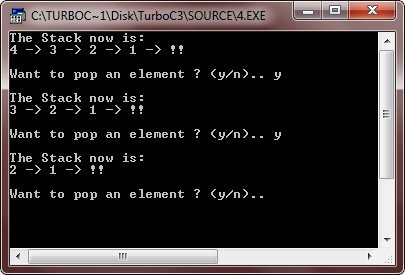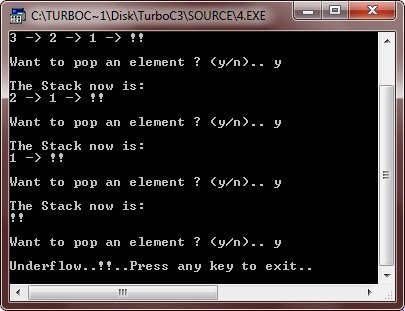Tools
Calculator